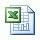# Starting volume of original mud (weight up with Barite)

You know how much volume will be increased due to adding barite into the system; however, you sometimes are limited to total volume due to limit pit volume on the rig so you need to calculate starting volume to achieve the predetermined final volume of desired mud weight. This formula below is used to determine the star volume of mud in case of weighting up with Barite.

Starting volume in bbl = VF x (35 – W2) ÷ (35 – W1)

Where; W1 = current mud weigth in ppg

W2 = new mud weight in ppg

VF = final volume in bbl

Example: Determine the starting volume (bbl) of 10.0 ppg (W1) mud required to achieve 100 bbl (VF) of 13.0 ppg (W2) mud with barite:

Starting volume in bbl = 100 x (35 – 13.0) ÷ (35 – 10.0)

Starting volume in bbl= 88.0 bbl

In order to achieve 100 bbl of 13.0 ppg mud weight up with barite, you must have 88.0 bbl starting volume of 10.0 ppg mud.

Please find the Excel sheetfor calculating Barrel of starting volume of original mud weight required to give a predetermined final volume of desired mud weight with barite.

Share the joy